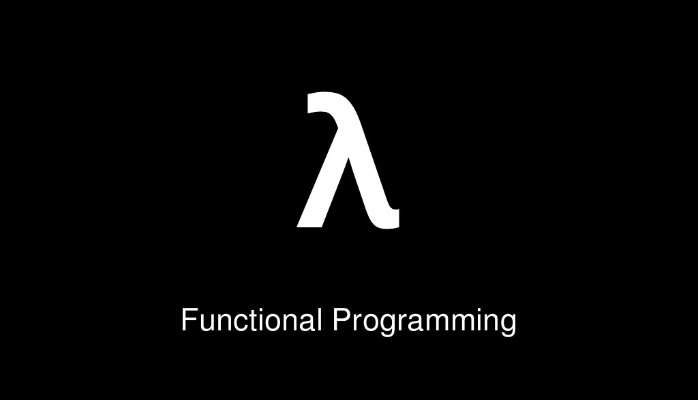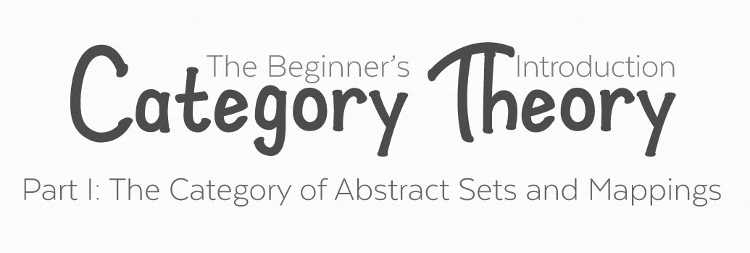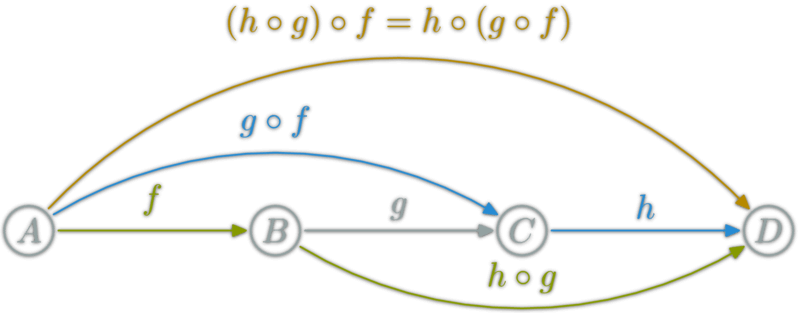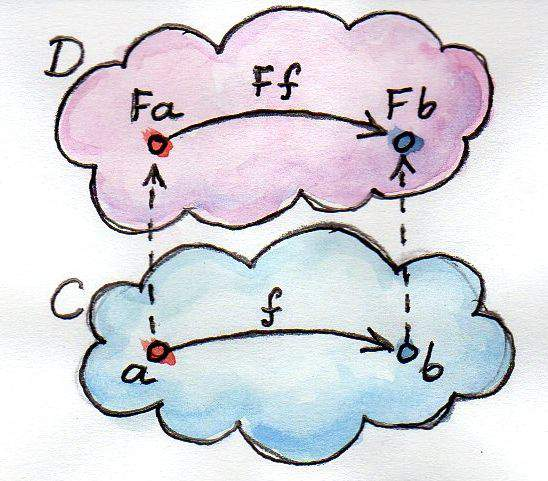# 函数式编程入门教程• 与面向对象编程（Object-oriented programming）和过程式编程（Procedural programming）并列的编程范式。
• 最主要的特征是，函数是第一等公民
• 强调将计算过程分解成可复用的函数，典型例子就是`map`方法和`reduce`方法组合而成 MapReduce 算法
• 只有纯的、没有副作用的函数，才是合格的函数。## 一、范畴论### 1.1 范畴的概念

"范畴就是使用箭头连接的物体。"（In mathematics, a category is an algebraic structure that comprises "objects" that are linked by "arrows". ）• 所有成员是一个集合
• 变形关系是函数

### 1.3 范畴与容器

• 值（value）
• 值的变形关系，也就是函数。

``````
class Category {
constructor(val) {
this.val = val;
}

return x + 1;
}
}
``````

## 二、函数的合成与柯里化

### 2.1 函数的合成``````
const compose = function (f, g) {
return function (x) {
return f(g(x));
};
}
````````````
compose(f, compose(g, h))
// 等同于
compose(compose(f, g), h)
// 等同于
compose(f, g, h)
``````

### 2.2 柯里化

`f(x)``g(x)`合成为`f(g(x))`，有一个隐藏的前提，就是`f``g`都只能接受一个参数。如果可以接受多个参数，比如`f(x, y)``g(a, b, c)`，函数合成就非常麻烦。

``````
// 柯里化之前
return x + y;
}

// 柯里化之后
return function (x) {
return x + y;
};
}

``````

## 三、函子

### 3.1 函子的概念### 3.2 函子的代码实现

``````
class Functor {
constructor(val) {
this.val = val;
}

map(f) {
return new Functor(f(this.val));
}
}
``````

``````
(new Functor(2)).map(function (two) {
return two + 2;
});
// Functor(4)

(new Functor('flamethrowers')).map(function(s) {
return s.toUpperCase();
});
// Functor('FLAMETHROWERS')

(new Functor('bombs')).map(_.concat(' away')).map(_.prop('length'));
// Functor(10)
``````

## 四、of 方法

``````
Functor.of = function(val) {
return new Functor(val);
};
``````

``````
Functor.of(2).map(function (two) {
return two + 2;
});
// Functor(4)
``````

## 五、Maybe 函子

``````
Functor.of(null).map(function (s) {
return s.toUpperCase();
});
// TypeError
``````

Maybe 函子就是为了解决这一类问题而设计的。简单说，它的`map`方法里面设置了空值检查。

``````
class Maybe extends Functor {
map(f) {
return this.val ? Maybe.of(f(this.val)) : Maybe.of(null);
}
}
``````

``````
Maybe.of(null).map(function (s) {
return s.toUpperCase();
});
// Maybe(null)
``````

## 六、Either 函子

Either 函子内部有两个值：左值（`Left`）和右值（`Right`）。右值是正常情况下使用的值，左值是右值不存在时使用的默认值。

``````
class Either extends Functor {
constructor(left, right) {
this.left = left;
this.right = right;
}

map(f) {
return this.right ?
Either.of(this.left, f(this.right)) :
Either.of(f(this.left), this.right);
}
}

Either.of = function (left, right) {
return new Either(left, right);
};
``````

``````
var addOne = function (x) {
return x + 1;
};

// Either(5, 7);

// Either(2, null);
``````

Either 函子的常见用途是提供默认值。下面是一个例子。

``````
Either
.map(updateField);
``````

Either 函子的另一个用途是代替`try...catch`，使用左值表示错误。

``````
function parseJSON(json) {
try {
return Either.of(null, JSON.parse(json));
} catch (e: Error) {
return Either.of(e, null);
}
}
``````

## 七、ap 函子

``````
return x + 2;
}

const A = Functor.of(2);
``````

ap 是 applicative（应用）的缩写。凡是部署了`ap`方法的函子，就是 ap 函子。

``````
class Ap extends Functor {
ap(F) {
return Ap.of(this.val(F.val));
}
}
``````

``````
// Ap(4)
``````

ap 函子的意义在于，对于那些多参数的函数，就可以从多个容器之中取值，实现函子的链式操作。

``````
return function (y) {
return x + y;
};
}

// Ap(5)
``````

``````
``````

``````
Maybe.of(
Maybe.of(
Maybe.of({name: 'Mulburry', number: 8402})
)
)
``````

Monad 函子的作用是，总是返回一个单层的函子。它有一个`flatMap`方法，与`map`方法作用相同，唯一的区别是如果生成了一个嵌套函子，它会取出后者内部的值，保证返回的永远是一个单层的容器，不会出现嵌套的情况。

``````
join() {
return this.val;
}
flatMap(f) {
return this.map(f).join();
}
}
``````

## 九、IO 操作

I/O 是不纯的操作，普通的函数式编程没法做，这时就需要把 IO 操作写成`Monad`函子，通过它来完成。

``````
var fs = require('fs');

return new IO(function() {
});
};

var print = function(x) {
return new IO(function() {
console.log(x);
return x;
});
}
``````

``````
.flatMap(print)
``````

``````
var tail = function(x) {
return new IO(function() {
return x[x.length - 1];
});
}

.flatMap(tail)
.flatMap(print)

// 等同于
.chain(tail)
.chain(print)
``````

## 十、参考链接

（正文完）

============================（完）

## 留言（75条）

@choukin，@yard：

@Parker Liu：

@阮一峰：

Category theory formalizes mathematical structure and its concepts in terms of a collection of objects and of arrows (also called morphisms). A category has two basic properties: the ability to compose the arrows associatively and the existence of an identity arrow for each object.

F id = id
F (f . g) = F f . F g

"箭头表示范畴成员之间的关系，正式的名称叫做"态射"（morphism）。范畴论认为，同一个范畴的所有成员，就是不同状态的"变形"（transformation）。通过"态射"，一个成员可以变形成另一个成员。"开头就有问题了. 范畴论的基础是不关心成员, 只考虑整体.

Java8的Lambda编程熟悉了,把你文章一看,理论知识更加丰富一层,赞!

// 柯里化之前
return x + y;
}

// 柯里化之后
return function (x) {
return x + y;
};
}

AP和Curry和像啊

@阮一峰老师：
2.1节函数的合成列子，根据上面的图我总感觉应该是如下：
const compose = function (f, g) {
return function (x) {
return g(f(x));
};
}

Maybe.of(null) 还是找不到toUpperCase() 继承没有使用重写的方法....
> node
> class Functor {
... constructor(val) {
..... this.val = val;
..... }
...
... map(f) {
... return new Functor(f(this.val));
... }
... }
[Function: Functor]
> Functor.of = function(val) {
... return new Functor(val);
... };
[Function]
> class Maybe extends Functor {
... map(f) {
..... return this.val ? Maybe.of(f(this.val)) : Maybe.of(null);
..... }
... }
[Function: Maybe]
> Maybe.of(null).map(function (s) {
... return s.toUpperCase();
... });
TypeError: Cannot read property 'toUpperCase' of null
at repl:2:9
at Functor.map (repl:7:20)
at repl:1:16
at ContextifyScript.Script.runInThisContext (vm.js:23:33)
at REPLServer.defaultEval (repl.js:334:29)
at bound (domain.js:280:14)
at REPLServer.runBound [as eval] (domain.js:293:12)
at REPLServer.onLine (repl.js:531:10)
at emitOne (events.js:101:20)
at REPLServer.emit (events.js:189:7)

2.1中为什么不是 return g(f(x));而是 return f(g(x))？

.flatMap(print)

return new IO(function() {
});
};

“2.1 函数的合成” 中，下面这段代码是不是错了？

const compose = function (f, g) {
return function (x) {
return f(g(x));
};
}

const compose = function (f, g) {
return function (x) {
return g(f(x));
};
}

@prettykernel：谢谢指出，已改正。

@cshenger：是的， Array 是函子。

@steam：两种写法都可以，函数式编程的惯例，似乎是先运行右边参数。

`引用动感小前端的发言：`

var curry = function (f) {
return function(x) {
return function(y) {
return f(x, y);
};
};
}

.flatMap(tail)
.flatMap(print)

@yybbb：

`(new Functor('bombs')).map(_.concat(' away')).map(_.prop('length'));`

- 我的环境下执行顺序是，
1.require('lodash')
2.执行代码

```
return new Functor(f(this.val));
^

TypeError: f is not a function
```

@yybbb：

class Functor {
constructor(val) {
this.val = val;
}
static of(val){
return new this(val)
}
map(f) {
return new Functor(f(this.val));
}
}

`引用聪明的剑圣的发言：`

@阮一峰老师：
2.1节函数的合成列子，根据上面的图我总感觉应该是如下：
const compose = function (f, g) {
return function (x) {
return g(f(x));
};
}

Either 函子这块

Either extends 了 Functor

transformation这个是“变换”的意思，线性代数里面我们就学过，线性变换linear transformation。

Either 函子定义错了，左值作为默认值，无论传递什么函数给它，它自身都不会改变。

class Either extends Functor {
constructor(left, right) {
this.left = left;
this.right = right;
}

map(f) {
return this.right ?
Either.of(this.left, f(this.right)) :
Either.of(this.left, this.right); //因为不做任何操作，这里也可以直接返回this
}
}

Either.of = function (left, right) {
return new Either(left, right);
};

Either用法 示例：
// Either("not valid number”, 7);

// Either("not valid number”, null); //右值无效的情况下左值作为默认值始终不变

@shinefine 说：

class Ap extends Functor {
ap(F) {
return Ap.of(this.val(F.val));
}
}

class Ap extends Functor {
ap(F) {
return Ap.of(F.map(this.val));
}
}

`引用sheng的发言：`

@shinefine 说：

@sheng

function IsOdd(n){
return n%2 ==0
}

var fs = require('fs');
var {IO} = require('./IO.js')

return new IO(function() {
}()//这个括号这里是改动的部分);
};

var print = function(x) {
return new IO(function() {
console.log(x);
return x;
}()//这个括号这里是改动的部分);
}

.flatMap(print)

`引用Parker Liu的发言：`

@阮一峰：

Category theory formalizes mathematical structure and its concepts in terms of a collection of objects and of arrows (also called morphisms). A category has two basic properties: the ability to compose the arrows associatively and the existence of an identity arrow for each object.

F id = id
F (f . g) = F f . F g

"函子只是Cat范畴中的态射，即Cat范畴（其对象是范畴）中的范畴之间的态射。" 谢谢指正，

IO单子示例部分“print”定义中“console.log(x)”似应为“console.log(x())”。

class Maybe extends Functor {
map(f) {
return this.val ? Maybe.of(f(this.val)) : Maybe.of(null);
}
}

Maybe.of(null).map(function (s) {
return s.toUpperCase();
});

const compose = function (f, g) {
return function (x) {
return f(g(x));
};
}

const add = function (c) {
return c+1;
}

const mult = function(t) {
return t * 2;
}

@yybbb：

IO IO IO IO 的代码呢？？？留一半不写全？？？？？
show me the code

«-必填

«-必填，不公开

«-我信任你，不会填写广告链接